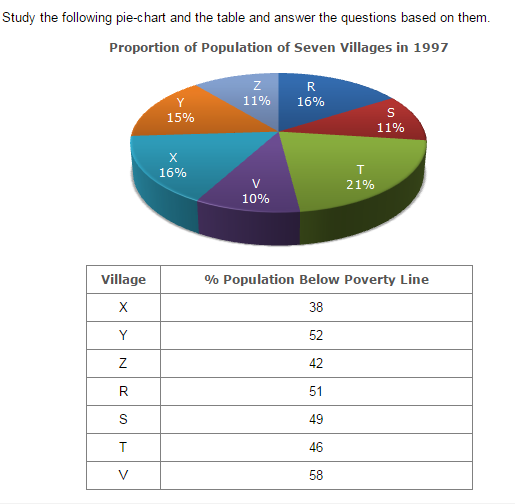Direction1.

Near about 20% of the funds are to be arranged through:

 A. SPVS B. External Assistance C. Annuity D. Market Borrowing
Answer:B
Explanation: 20% of the total funds to be arranged = Rs. (20% of 57600) crores = Rs. 11520 crores ~= Rs. 11486 crores. Rs. 11486 crores is the amount of funds to be arranged through External Assistance.

2.

If NHAI could receive a total of Rs. 9695 crores as External Assistance, by what percent (approximately) should it increase the Market Borrowing to arrange for the shortage of funds?

 A. 4.5% B. 7.5% C. 6% D. 8%
Answer:C
Explanation: Shortage of funds arranged through External Assistance Therefore = Rs. (11486 - 9695) crores = Rs. 1791 crores. Therefore Increase required in Market Borrowing = Rs. 1791 crores. Percentage increase required = ( 1791 x 100 ) % = 5.98% ~= 6%. 29952

3.

If the toll is to be collected through an outsourced agency by allowing a maximum 10% commission, how much amount should be permitted to be collected by the outsourced agency, so that the project is supported with Rs. 4910 crores?

 A. Rs. 6213 crores B. Rs. 5827 crores C. Rs. 5401 crores D. Rs. 5316 crores
Answer:C
Explanation: Amount permitted = (Funds required from Toll for projects of Phase II) + (10% of these funds) = Rs. 4910 crores + Rs. (10% of 4910) crores = Rs. (4910 + 491) crores = Rs. 5401 crores.

4.

The central angle corresponding to Market Borrowing is

 A. 52º B. 137.8º C. 187.2º D. 192.4º
Answer:C
Explanation: Central angle corresponding to Market Borrowing = ( 29952 x 360º ( = 187.2º 57600

5.

The approximate ratio of the funds to be arranged through Toll and that through Market Borrowing is

 A. 2 : 9 B. 1 : 6 C. 3 : 11 D. 2 : 5
Answer:B
Explanation: Required ratio = 4910 = 1 ~= 1 . 29952 6.1 6